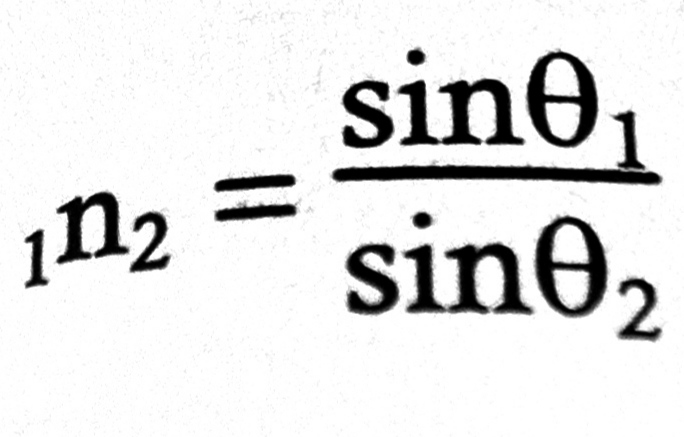Refraction of light (introduction)﻿(here the term n is read as muon)

Refraction of light

In a homogeneous medium, light travels along a straight path. But when a ray of light travels from one transparent medium to another, it bends while crossing the surface separating the two media. The phenomenon of bending of light as it goes from one medium to another is called refraction of light.The angle between the incident ray and the normal denoted by 'i' is called angle of incidence, while the angle between refracted ray and the normal denoted by 'r' is called angle of refraction.

Laws of refraction

First law

The incident ray, the refracted ray and the normal at the point of incidence all lie in the same plane.

Second law

The sine of the angle of incidence and the sine of the angle of refraction bears a constant ratio, called the refractive index of the second medium with respect to the first medium. MathematicallyThis is called snell's law.

Absolute refractive index

The absolute refractive index of a medium is defined as the ratio of the velocity of the light in vaccum to the velocity of the light in medium. That is,Where, c=3.0×108is the velocity of the light in the vaccum.

Relative Refractive index (1n2)

The relative refractive index of the second medium with respect to the first medium is defined as the ratio of the velocity of the light in the first medium to the velocity of the light in the second medium. That is,Where v1 and v2 are the velocities of light in the fist and the second medium respectively.

Relation between refractive indices

1. A ray of light AO is passing from medium 1 to medium 2, which travels along OB after refraction at O. Let θ1 be the angle of incidence in medium 1 and θ 2 be the angle of refraction in medium 2. Then, refractive index for light from medium 1 to 2 is given byAccording to the principle of reversibility of light, it follows that a ray traveling along BO in medium 2 is refracted along OA in medium 1. Hence refractive index from medium 2 to medium 1,Thus, if medium 1 is air, medium 2 is water and the refractive index from air to water is 3/4 then that from water to air is 4/3.2. A ray if light is traveling from medium 1 to medium 2 to medium 3 to medium 1. We consider air as medium 1, water as medium 2, and glass as medium 3. If the boundaries of the media are parallel, experiment shows that the emergent ray is parallel to the incident ray, although it is laterally displaced. Suppose the incident ray AB is refracted from the medium 1 into medium 2 along BC, from medium 2 into medium 3 along CD and from medium 3 into medium 1 along DE. Since AB and DE are parallel to eachother, the angle made by AB with normal at B is equal to the angle made by DE with the normal at D. Let us denote them by θ1. Let θ2 and  θ3 are the angles made by the respective rays in medium 2 and medium 3 with the normals at B and D respectively.The formula 1n× 2n31n3 can easily be recalled with the help of the order of the subscripts. For example, suppose medium 1,2 and 3 are respeectively air, water, and glass and we wish to know the refractive index from water to glass (wng). If we know refractive index from air to water (anw) and refractive index from air to glass (ang), then.3. A ray of light AO is traveling from medium 1 to medium 2. It is refracted into the medium 2 along OB. Let θ1 and θ2 be the angles made by the rays AO and OB with the normal at O. From Snell's law,n1 and n2 are the absolute refractive indices of medium 1 and medium 2 respectively.This relation shows that, when a ray is refracted from one medium to another, the boundaries being parallel,

n sinθ = constant.